# Errata: In Example 8.8, explanation of effects of poles and zeros is incorrect and confusing

Location: page 254, line -10 to page 255, line 9

In the description of the gain and phase curves in Example 8.8, there are multiple errors:

• The breakpoint frequencies should use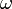instead of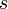in the text and in Figure 8.14;
• The frequencies where the breakpoints occur are not the locations of the poles and zeros. It is the effects of the poles and zeros that is relevant.

The correct text for the last two paragraphs of the exercise is given below:

We begin with the gain curve. At low frequency, the magnitude is given by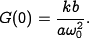When we reach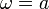, the effect of the pole begins and the gain decreases with slope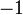. At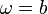, the zero comes into play and we increase the slope by 1, leaving the asymptote with net slope 0. This slope is used until the effect of the second-order pole is seen at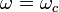, at which point the asymptote changes to slope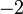. We see that the gain curve is fairly accurate except in the region of the peak due to the second-order pole (since for this case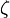is reasonably small).

The phase curve is more complicated since the effect of the phase stretches out much further. The effect of the pole begins at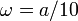, at which point we change from phase 0 to a slope of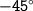/decade. The zero begins to affect the phase at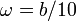, producing a flat section in the phase. At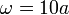the phase contributions from the pole end, and we are left with a slope of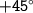/decade (from the zero). At the location of the second-order pole,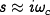, we get a jump in phase of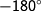. Finally, at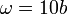the phase contributions of the zero end, and we are left with a phase of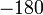degrees. We see that the straight-line approximation for the phase is not as accurate as it was for the gain curve, but it does capture the basic features of the phase changes as a function of frequency.

The correct version of Figure 8.14 is(Contributed by L. Xiong, 29 Dec 08)